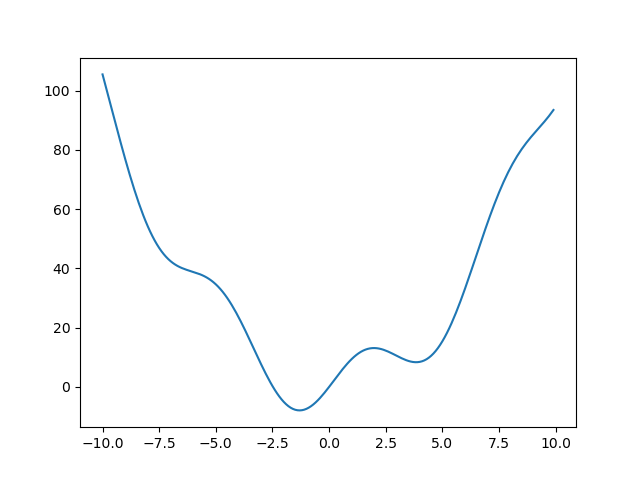# 1.6.12.1. Finding the minimum of a smooth function¶

Demos various methods to find the minimum of a function.

```import numpy as np
import matplotlib.pyplot as plt

def f(x):
return x**2 + 10*np.sin(x)

x = np.arange(-10, 10, 0.1)
plt.plot(x, f(x))
```Now find the minimum with a few methods

```from scipy import optimize

print(optimize.minimize(f, x0=0))
```

Out:

```fun: -7.945823375615215
hess_inv: array([[0.08589237]])
jac: array([-1.1920929e-06])
message: 'Optimization terminated successfully.'
nfev: 18
nit: 5
njev: 6
status: 0
success: True
x: array([-1.30644012])
```
```print(optimize.minimize(f, x0=0, method="L-BFGS-B"))
```

Out:

```fun: array([-7.94582338])
hess_inv: <1x1 LbfgsInvHessProduct with dtype=float64>
jac: array([-1.42108547e-06])
```plt.show()